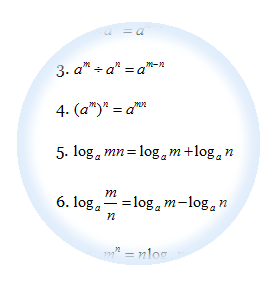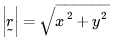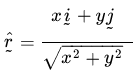Formulae that will be given in SPM Add MathsThese are the formulae that will be given to help you to answer the SPM Additional Maths Questions. However, more important is that you know how to apply those formulaes to solve your questions. Buy some exercises from the bookstore and work on the questions everyday and you will master all those formulaes. Basically there are divided into 5 categories:

1. Algebra
2. Calculus
3. Statistics
4. Geometry
5. Trigonometry

Algebra

1.$x=\frac{-b\pm \sqrt{{{b}^{2}}-4ac}}{2a}$

2.${{a}^{m}}\times {{a}^{n}}={{a}^{m+n}}$

3.${{a}^{m}}\div {{a}^{n}}={{a}^{m-n}}$

4.${{({{a}^{m}})}^{n}}={{a}^{mn}}$

5.${{\log }_{a}}mn={{\log }_{a}}m+{{\log }_{a}}n$

6.${{\log }_{a}}\frac{m}{n}={{\log }_{a}}m-{{\log }_{a}}n$

7.${{\log }_{a}}{{m}^{n}}=n{{\log }_{a}}m$

8.${{\log }_{a}}b=\frac{{{\log }_{c}}b}{{{\log }_{c}}a}$

9.${{T}_{n}}=a+(n-1)d$

10.${{S}_{n}}=\frac{n}{2}\left[ 2a+(n-1)d \right]$

11.${{T}_{n}}=a{{r}^{n-1}}$

12.${{S}_{n}}=\frac{a({{r}^{n}}-1)}{r-1}=\frac{a(1-{{r}^{n}})}{1-r},r\ne 1$

13.${{S}_{\infty }}=\frac{a}{1-r},\left| r \right|<1$

Calculus

1.$y=uv,\frac{dy}{dx}=u\frac{dv}{dx}+v\frac{du}{dx}$

2.$y=\frac{u}{v},\frac{dy}{dx}=\frac{v\frac{du}{dx}-u\frac{dv}{dx}}{{{v}^{2}}}$

3.$\frac{dy}{dx}=\frac{dy}{du}\times \frac{du}{dx}$

4. Area under a curve =$\int\limits_{a}^{b}{y}\text{ }dx\text{ or }\int\limits_{a}^{b}{x}\text{ }dy$

5. Volume generated =$\int\limits_{a}^{b}{\pi {{y}^{2}}}\text{ }dx\text{ or }\int\limits_{a}^{b}{\pi {{x}^{2}}}\text{ }dy$

Statistics

1.$\overline{x}=\frac{\sum{x}}{N}$

2.$\overline{x}=\frac{\sum{fx}}{\sum{f}}$

3.$\sigma =\sqrt{\frac{\sum{{{(x-\overline{x})}^{2}}}}{N}}={{\sqrt{\frac{\sum{{{x}^{2}}}}{N}-{{\overline{x}}^{2}}}}^{{}}}$

4.$\sigma =\sqrt{\frac{\sum{f{{(x-\overline{x})}^{2}}}}{\sum{f}}}=\sqrt{\frac{\sum{f{{x}^{2}}}}{\sum{f}}-{{\overline{x}}^{2}}}$

5.$m=L+\left( \frac{\frac{1}{2}N-F}{{{f}_{m}}} \right)C$

6.$I=\frac{{{Q}_{1}}}{{{Q}_{0}}}\times 100$

7.$\overline{I}=\frac{\sum{{{W}_{i}}{{I}_{i}}}}{\sum{{{W}_{i}}}}$

8.${}^{n}{{\text{P}}_{r}}=\frac{n!}{(n-r)!}$

9.${}^{n}{{C}_{r}}=\frac{n!}{(n-r)!r!}$

10.$P(A\cup B)=P\left( A \right)+P\left( B \right)-P(A\cap B)$

11.$P(X=r)={}^{n}{{C}_{r}}{{p}^{r}}{{q}^{n-r}},\text{ where }p+q=1$

12. Mean/Min$\mu =np$

13.$\sigma =\sqrt{npq}$

14.$Z=\frac{X-\mu }{\sigma }$

Geometry

1. Distance =$\sqrt{{{\left( {{x}_{2}}-{{x}_{1}} \right)}^{2}}+{{\left( {{y}_{2}}-{{y}_{1}} \right)}^{2}}}$

2. Midpoint$(x,y)=\left( \frac{{{x}_{1}}+{{x}_{2}}}{2},\frac{{{y}_{1}}+{{y}_{2}}}{2} \right)$

3. A point dividing a segment of a line$(x,y)=\left( \frac{n{{x}_{1}}+m{{x}_{2}}}{m+n},\frac{n{{y}_{1}}+m{{y}_{2}}}{m+n} \right)$

4. Area of a triangle =$\frac{1}{2}\left| ({{x}_{1}}{{y}_{2}}+{{x}_{2}}{{y}_{3}}+{{x}_{3}}{{y}_{1}})-({{x}_{2}}{{y}_{1}}+{{x}_{3}}{{y}_{2}}+{{x}_{1}}{{y}_{3}}) \right|$

5.6.Trigonometry

1. Length of an arc,$s=r\theta$

2. Area of a sector,$A=\frac{1}{2}{{r}^{2}}\theta$

3.${{\sin }^{2}}A+{{\cos }^{2}}A=1$

4.${{\sec }^{2}}A=1+{{\tan }^{2}}A$

5.$co{{\sec}^{2}}A=1+{{\cot }^{2}}A$

6.$\sin 2A=2\sin A\cos A$

7.$\begin{array}{rcl} \cos 2A & = & {{\cos }^{2}}A-{{\sin }^{2}}A \\ & = & 2{{\cos }^{2}}A-1 \\ & = & 1-2{{\sin }^{2}}A \end{array}$

8.$\sin \left( A\pm B \right)=\sin A\cos B\pm \cos A\sin B$

9.$\cos \left( A\pm B \right)=\cos A\cos B\mp \sin A\sin B$

10.$\tan \left( A\pm B \right)=\frac{\tan A\pm \tan B}{1\mp \tan A\tan B}$

11.$\tan 2A=\frac{2\tan A}{1-{{\tan }^{2}}A}$

12.$\frac{a}{\sin A}=\frac{b}{\sin B}=\frac{c}{\sin C}$

13.${{a}^{2}}={{b}^{2}}+{{c}^{2}}-2bc\cos A$

14. Area of a triangle =$\frac{1}{2}ab\sin C$

3 thoughts on “Formulae that will be given in SPM Add Maths”

1.Erisa says:

for the algebra, is it algebra 1 or 2 or 3? or all 3?

1.Mr Low says:

This is just the exact formulae sheet that will be given in spm exam.

2.[email protected] says:

It is very useful

This site uses Akismet to reduce spam. Learn how your comment data is processed.